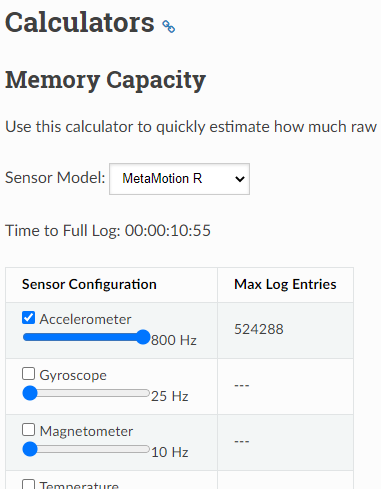# Max logging data size

Hello,

I have MetaMotionR. Could you please let me know what is the max size of the data that can be stored in MetaMotionR for logging (not streaming)?
In the MetaMotionR datasheet, it says "512 kB flash program memory, 64 kB RAM).
Does this mean that the max size of measured and stored data is 64kB for logging?

Thanks.

• See our tutorials calculator section

• @Laura said:
See our tutorials calculator section

Hello,

Thanks for pointing the site.
I calculated the max log entry for 800Hz sampling with accelerometer selected (no other sensor selected).
Then, it gives 524kB for max log entry (pl. see the attached screenshot below)

But, I tried recording (log) 1 min data with 800Hz (acceleration data only) and it seems to work. The logged data (sent via email) is ~2MB.

How could I get larger logged data of ~2MB than the possible data size of 524kB? Should I not trust longer logged data than max log entry (524kB)?Thanks.

• Hi Laura,
So, how should we estimate correct data size for given sampling rate?
MetaMotionR sends larger data (2MB) than the estimate (524kB) because MetaMotionR uses a combination of both logging and streaming at the same time even though we set up to log only?

Thanks.

• That's not 524Kb, that's the number of samples. 524288 data samples

• Hi Laura,

I see. Thanks for the clarification. I was wrong that I thought it was in byte. Now I know that it is the number of entries.

• Is each number counted toward 524288, so one sampling (time=1, x=1, Y=1, z=1, total 4) counted as FOUR entries?
Or is one sampling (time=1, x=1, Y=1, z=1, total 4) counted as ONE toward 524288?

• Also, the data size for 524288 entries (one sampling is counted as ONE) is ~23MB.Is the logged data stored in the data RAM which is only 64kB?

Thanks.

• (time=1, x=1, Y=1, z=1, total 4) is counter as ONE ENTRY
The data is stored in the NOR flash in a custom way (don't worry too much about figuring out size).